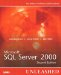# Evaluating Index Usefulness

SQL Server provides indexes for two primary reasons: as a method to enforce the uniqueness of the data in the database tables and to provide faster access to data in the tables. Creating the appropriate indexes for a database is one of the most important aspects of your physical database design. Because you can't have an unlimited number of indexes on a table, and it wouldn't be feasible anyway, you'll want to create indexes on columns that have high selectivity so that the index will be used by your queries. The selectivity of an index can be defined as follows :

Selectivity ratio = (Number of unique index values)/ (Total number of rows in the table)

If the selectivity ratio is high ”that is, a large number of rows can be uniquely identified by the key ”then the index is highly selective and useful to the optimizer. The optimum selectivity would be 1, meaning that there is a unique value for each row. A low selectivity means that there are many duplicate values and the index would be less useful. The SQL Server optimizer decides whether to use any indexes for a query based on the selectivity of the index. The higher the selectivity, the faster and more efficiently SQL Server can retrieve the resultset.

For example, say that you are evaluating useful indexes on the authors table. Assume that most of the queries access the table either by the author's last name or by state. Because a large number of concurrent users modify data in this table, you are allowed to choose only one index ”author's last name or state. Which one should you choose? Let's perform some analysis to see which one is a more useful, or selective, index. First, determine the selectivity based on the author's last name with a query on the authors table in the pubs database:

` select count(distinct au_lname) as '# unique',     count(*) as '# rows',    str(count(distinct au_lname) / cast (count(*) as real),4,2) as 'selectivity' from authors go # unique    # rows      selectivity ----------- ----------- -----------          22          23 0.96 `

The selectivity ratio calculated for the au_lname column on the authors table, 0.96, indicates that an index on au_lnam e would be highly selective and a good candidate for an index. All rows but one in the table contain a unique value for last name.

Now, look at the selectivity of the state column:

` select count(distinct state) as '# unique',      count(*) '# rows',     str(count(distinct state) / cast (count(*) as real),4,2) as 'selectivity' from authors go # unique    # rows      selectivity ----------- ----------- -----------           8          23 0.35 `

As you can see, an index on the state column would be much less selective (0.35) than an index on the au_lname column and possibly not as useful.

One of the questions to ask at this point is whether a few values in the state column that have a high number of duplicates are skewing the selectivity, or whether there are just a few unique values in the table. You can determine this with a query similar to the following:

` select state, count(*)  from authors group by state order by 2 desc go state ----- ----------- CA             15 UT              2 TN              1 MI              1 OR              1 IN              1 KS              1 MD              1 `

As you can see, the state values are relatively unique, except for one. More than half the rows in the table have the same value of ' CA ' for state . Therefore, state is probably not a good candidate for an indexed column, especially if most of the time you are searching for authors from the state of California. SQL Server would generally find it more efficient to scan the whole table rather than search via the index.

Generally, if the selectivity ratio for a key is less than .85 (in other words, the optimizer cannot discard at least 85 percent of the rows based on the key value), then the optimizer generally chooses a table scan to process the query. In such cases, performing a table scan to find all the qualifying rows is more efficient than seeking through the B-tree to locate a large number of data rows.

## NOTE

You can relate the concept of selectivity to a somewhat real-world example. What if you had to find every instance of the words "SQL Server" in this book? Would it be easier to do it by using the index and going back and forth from the index to all the pages that contain the words, or would it be easier just to scan each page from beginning to end to locate them? What if you had to find all references to the word "Squonk," if any? Squonk would definitely be easier to find via the index (actually the index would help you determine that it doesn't even exist). Therefore, the selectivity for "Squonk" would be high, and the selectivity for "SQL Server" would be much lower.

How does SQL Server determine whether an index is selective and which index, if it has more than one to choose from, would be the most efficient to use? For example, how would SQL Server know how many rows might be returned by the following query?

` select * from table       where key between 1000000 and 2000000 `

If the table contains 10,000,000 rows with values ranging between 0 and 20,000,000, how does the optimizer know whether to use an index or table scan? There could be 10 rows in the range, or 900,000. How does SQL Server estimate how many rows are between 1,000,000 and 2,000,000? The optimizer gets this information from the index statistics, as described in the next section.Microsoft SQL Server 2000 Unleashed (2nd Edition)
ISBN: 0672324679
EAN: 2147483647
Year: 2002
Pages: 503

Similar book on Amazon